### Home > PC > Chapter 4 > Lesson 4.2.3 > Problem4-98

4-98.
1. Simplify the following trig expressions. Homework Help ✎

1.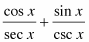2.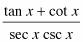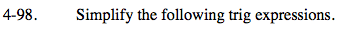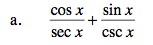1. Change the sec x and csc x to forms of the sine and cosine.
2. Simplify.
3. Substitute using the Fundamental Pythagorean Identities.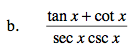$\frac{\frac{\text{sin}x)}{\text{cos}(x)}+\frac{\text{cos}(x)}{\text{sin}(x)}}{\text{sec}(x)\text{csc}(x)}$

$\small \frac{\frac{\text{sin}^2(x)+\text{cos}^2(x)}{\text{cos}(x)\text{sin}(x)}}{\frac{1}{\text{cos}(x)sin(x)}}=1$

$\small\frac{\frac{1}{\text{cos}(x)\text{sin}(x)}}{\frac{1}{\text{cos}(x)\text{sin}(x)}}=1$